# Detection of Duplicate Records in Large-scale Multi-tenant Platforms#PyConIE - 6th November 2016

## ME:

Currently Data Scientist at ...Twitter: @paul_ogrady

## Overview• Multi-Tenant Platforms
• Cartesian Joins
• Locality Sensitive Hashing
• LSH tuning

## Platform• The task of finding records in a data set that refer to the same entity across different data sources

• Necessary when joining data sets based on entities that may or may not share a common identifier—canonical ID

• Common problem, e.g.:

+----+------------+-------------+---------------------------------+
| ID | First Name | Second Name | Address                         |
+----+------------+-------------+---------------------------------+
| 19 | G.         | Van Rossum  | PSF, 9450 SW Gemini Dr. ...     |
+----+------------+-------------+---------------------------------+
| 56 | Guido      | V. Rossum   | Python Software Foundation, ... |
+----+------------+-------------+---------------------------------+


## Multi-Tenant Platform

Records $$\equiv$$ Products

• One platform, multiple (siloed) users may be selling the same products leading to duplicates
• Unique IDs may be unreliable, therefore we examine record content, i.e., product attributes
• Grouping duplicates under a canonical ID helps with:
• Satisfying customer requests (Smart Logistics)
• BI & ML insights (Smart Product Platform)
• Cross-sectional insights (How products are bought)

## Product Similarity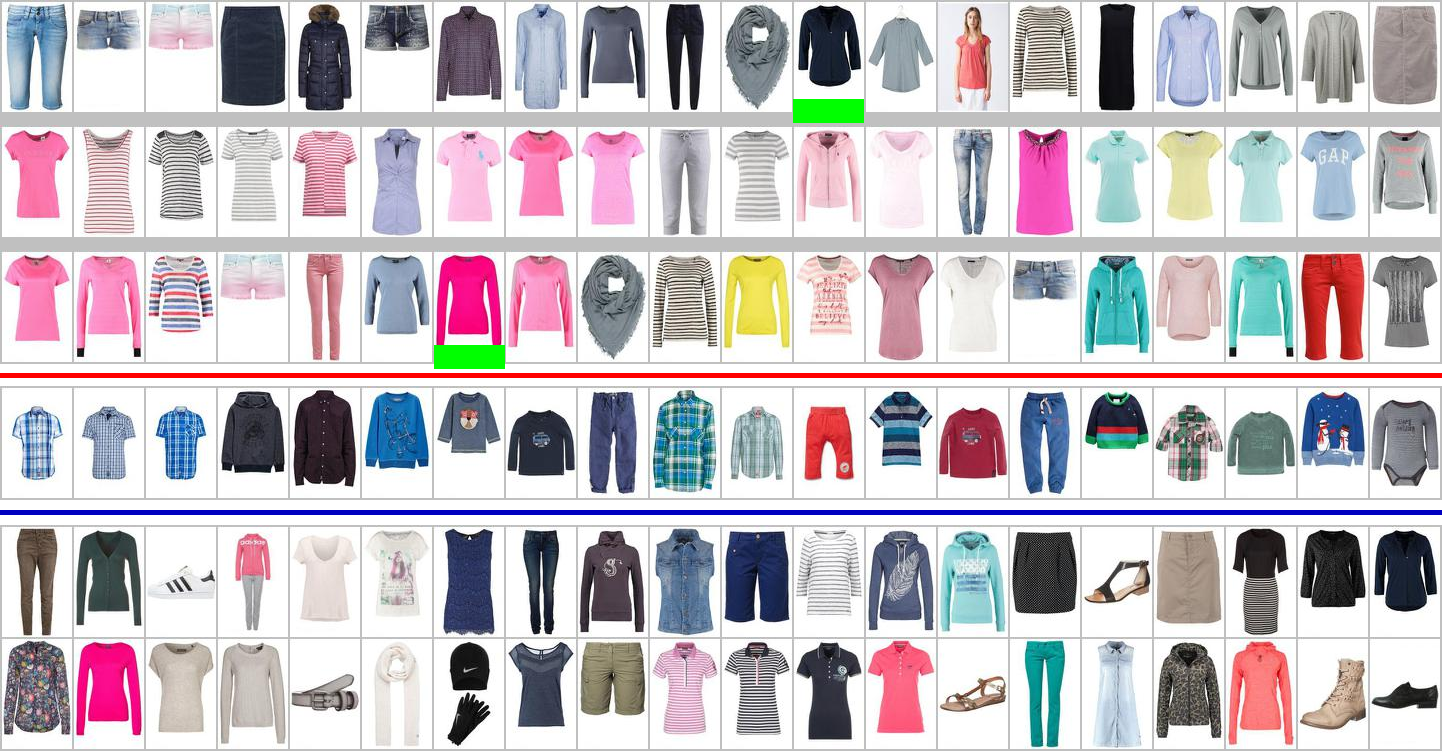## Product Similarity

• Record Linkage can be tackled using a notion of record similarity, where duplicates are close together
• Product attributes may have ordinal or categorical values, or contain images
• Ordinal Data: Vector space, cosine similarity, TFIDF
• Categorical Data: No order, cosine similarity will not work here
• Image Data: Feature Vectors, Deep Learning

We will be looking at Categorical Data

## Product Space

Products exist in space/manifold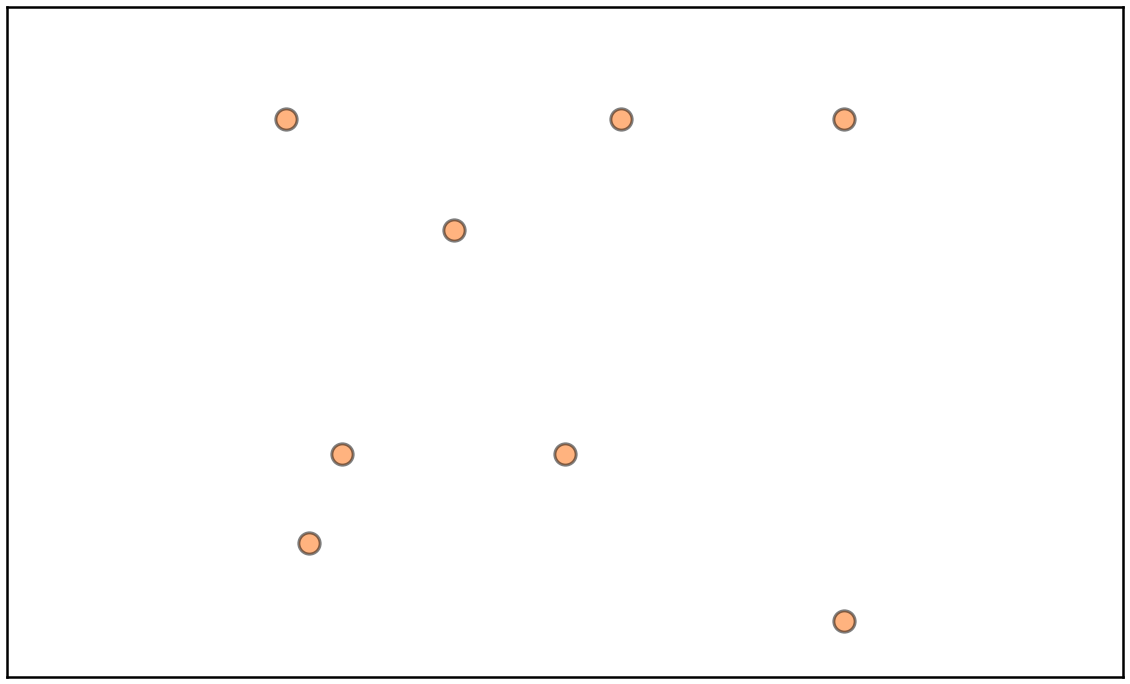## Product Space

Distances between products indicate similarity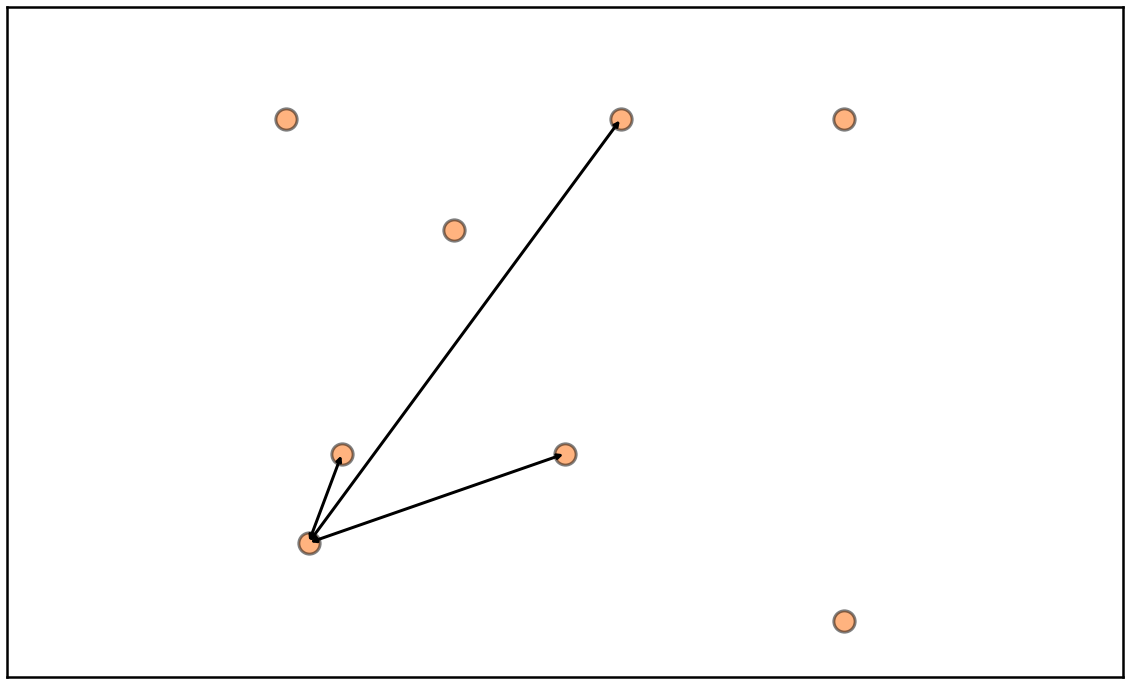## Product Space

Product duplicates are products that are very similar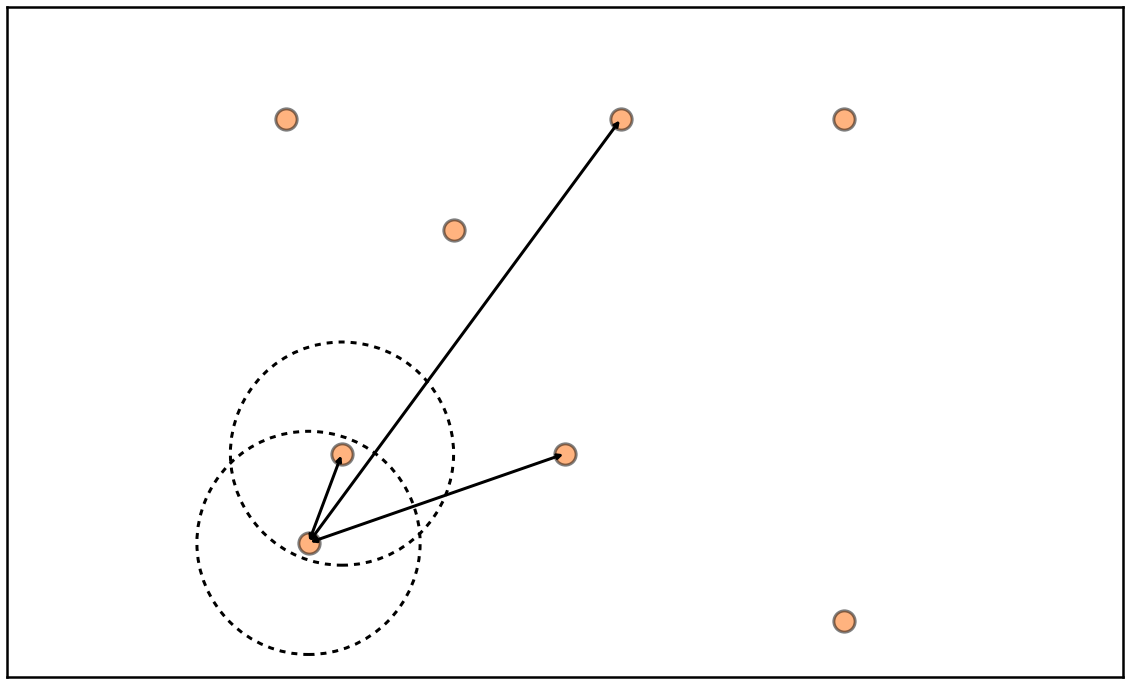## Cartesian Join

• Cartesian Join / All-pairs Join / Similarity Join $$O(n^2)$$ *

• A 10-fold increase in the input results in a 100-fold increase in the output—grows quadratically!
• Naive Solution:

sim_scores = []
for i, record_i  in enumerate(records):
for j, record_j in enumerate(records):
sim_scores.append(((i, j), sim(record_i, record_j)))

• Output:

>>> sim_scores
[((0, 0), 0), ((0, 1), 4), ((0, 2), 0), ((1, 0), -4), ((1, 1), 0),
((1, 2), -4), ((2, 0), 0), ((2, 1), 4), ((2, 2), 0)]


* To be more correct our problem is O(n(n-1)/2)

## Measuring Similarity

• How to calculate sim(record_i, record_j)?

• Composite Similarity Measure:

Weighted combination of individual attribute similarity measures, $${f_i,\dots,f_N}$$:

$\begin{split}S(p_i, p_j) & = w_1 f_1(a_{i1}, a_{j1}) + w_2 f_2(a_{i2}, a_{j2}) \\ & + \dots + w_N f_N(a_{iN}, a_{jN})\end{split}$

where $${a_i,\dots,a_N}$$ are the product attributes, $${f_i,\dots,f_N}$$ are their similarity measures and $${w_i,\dots,w_N}$$ are the weighting in the model .

## Composite Similarity Measure

• $$f_i$$ we use Hamming Distance between attributes *

• $$w_i$$ are learned from training data using an Averaged Perceptron, $$\hat y = \text{sign}(\mathbf{w_{avg}} \cdot \mathbf{x})$$

• Code:

for t in range(T):
for i in range(M):
x = training_data[i,:] # f() applied in advance
y = labels[i]
dot_prod = float(w.dot(np.matrix(x).T))
y_est = sgn(dot_prod)
if y_est != y:
w = w + (y * x)
w_avg += w

w_avg = w_avg / (T*M)


* We use other categorical similarity measures that we do not discuss here

## Hamming Distance

• The Hamming/Overlap distance between two records is the number of attributes in which they differ

$f_i(x, y) = H(x,y) = \sum_{i=1}^{N} \text{int}(x_i \neq y_i)$
• Code:

def hamming_distance(x, y):
return sum(elx != ely for elx, ely in zip(x, y))

• Output:

>>> x = "Foo Bar Baz Qux"; y = "Foo Bar Baz corge"
>>> hamming_distance(x, y)
1


## Scaling Up## Scaling Up De-duplication

• Problem grows quadratically; quickly run into trouble as # of Products > 1M (499,999,500,000 comparisons)
• All-pairs similarity calculates all scores, misses no duplicates

Q: If we relax our requirements on missing duplicates can we use a trick?

A: Yes - Locality-Sensitive Hashing (LSH)

## Locality-Sensitive Hashing

• LSH hashes input items so that similar items map to the same buckets with high probability 

• LSH differs from cryptography hash functions because it aims to maximize the probability of collisions

• Similarity is measured using the Jaccard Index

• LSH parameters:

• $$r$$: number of rows/hashes per band
• $$b$$: number of bands

LSH is an exercise in tuning r & b for desired similarity & accuracy

## Jaccard Index

• The Jaccard index is useful for comparing segments of text

$J(x,y) = {{|x \cap y|}\over{|x \cup y|}} = {{|x \cap y|}\over{|x| + |y| - |x \cap y|}}$
• Code:

def jaccard_index(x, y):
return len(x.intersection(y)) / len(x.union(y))

• Example:

>>> x = "Foo Bar Baz Qux"; y = "Qux Baz Bar Quux"
>>> jaccard_index(set(x.split()), set(y.split()))
0.6


## Simple Hashing

• Simple hashing function that hashes $$x$$ to $$N$$ buckets:

$h_{a}(x) = (a x + 1) \, \text{mod} \, N$

where $$a$$ is a random integer & $$N$$ is a prime number (to avoid collisions)

• Code:

class SimpleHash:
def __init__(self, a, N, c=1):
self.a = a; self.N = N; self.c = c
def __call__(self, x):
return (self.a * x + self.c)  % self.N

• Example:

>>> hash_ = SimpleHash(a=5, N=7)
>>> [hash_(i) for i in range(7)]
[1, 6, 4, 2, 0, 5, 3]


## Min-Hashing

• Convert large sets to short signatures, while preserving similarity

$Pr[h_\pi(p_1) = h_\pi (p_2)] = J(p_1, p_2);$

For a random permutation $$\pi$$.

• Code:

def min_hash(a, hash_func):
perm_ind = [hash_func(x) for x in range(a.shape)]

• Example:

>>> record_elements = np.array([0, 0, 1, 1, 0, 1, 1] )
>>> hash_ = simple_hash(a=5, N=7); [hash_(i) for i in range(7)]
[1, 6, 4, 2, 0, 5, 3]
>>> min_hash(record_elements, hash_)
2


## Min-Hashing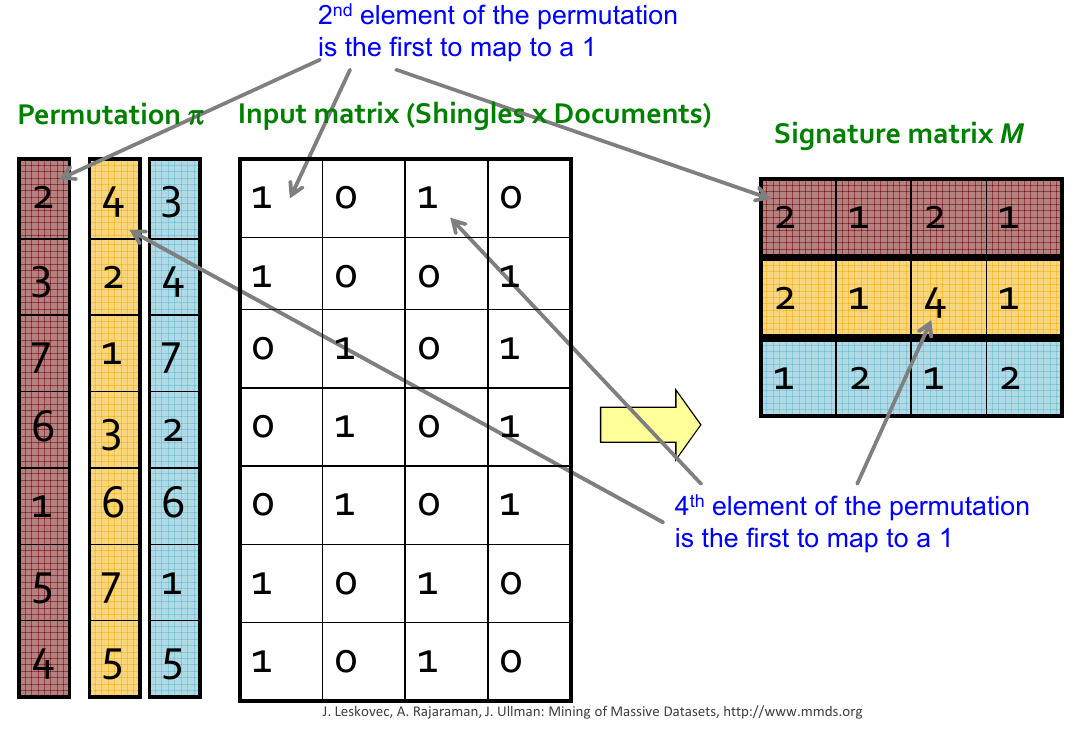## LSH Band Hashing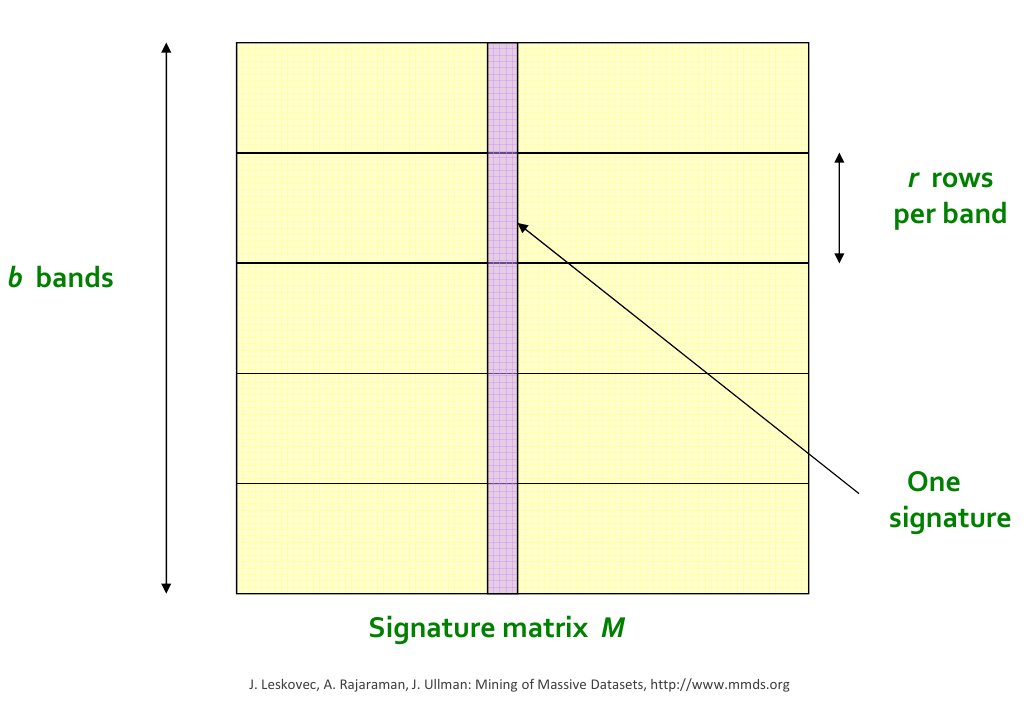## LSH Bucketing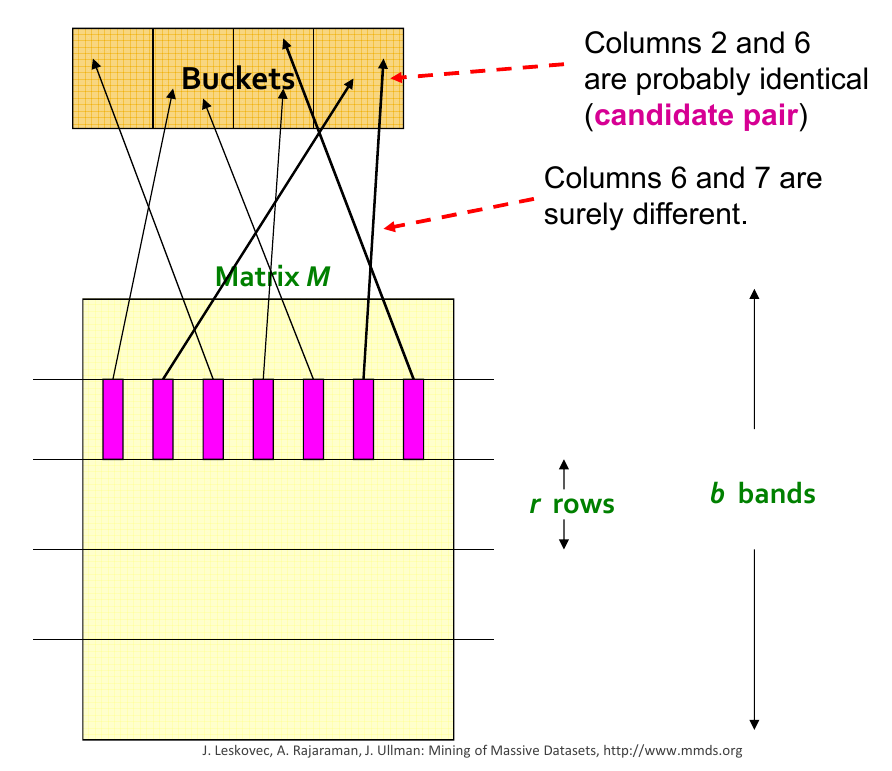## LSH Duplicate Detection

• Depending on $$r$$ & $$b$$ documents in buckets can be considered duplicate candidates
• A subsequent exact comparison is performed on candidates to identify duplicates
• Cartesian join on Candidates
• Composite Similarity Measure
• We use LSH as a duplicate pre-filtering step, then follow with an exact comparison between candidates

## System Tuning## Prob. of Two Products in Same Bucket?

For s-similar products, where r is the number of rows and b is the number of bands

$t = \operatorname{jaccard}(P_1, P_2)$

prob. that all rows in band equal, $$t^r$$

prob. that some row in band unequal, $$1 - t^r$$

prob. that no band identical, $$(1 - t^r)^b$$

prob. that at least 1 band identical (s-curve),

$1 - (1 - t^r)^b.$

## LSH S-curve Tuning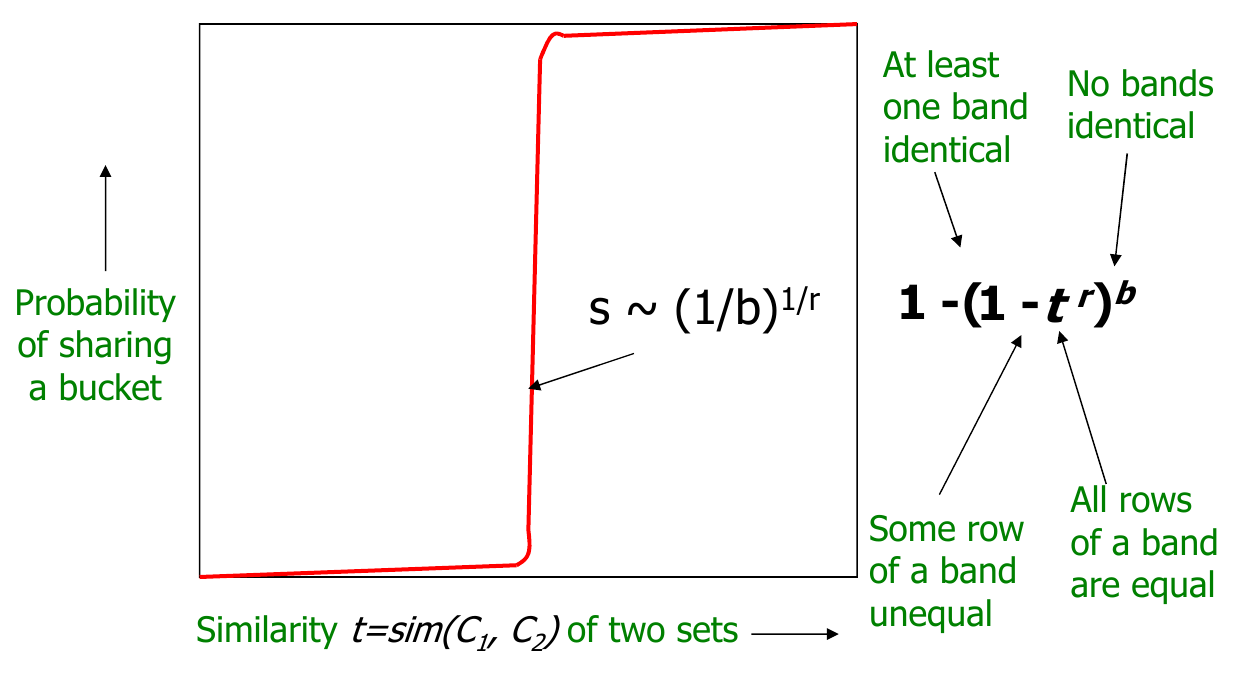## Candidate-Pair Probability

• LSH params: $$r=4$$ & $$b=16$$. Candidate Pair Probability:

Similarity Probability
0.00 0.000000000
0.10 0.001598801
0.20 0.025295082
0.30 0.122016723
0.40 0.339616210
0.50 0.643925870
0.60 0.891481916
0.70 0.987637591
0.80 0.999782058
0.90 0.999999962

## Tuning r & b for specified s-similarity

• Optimise $$1 - (1 - t^r)^b$$

• Reduce the area on the left of the s-curve threshold
• Reduce the distance between the s-curve threshold and a specified target
• How to optimise?

• Calc. max of derivative of candidate prob using sympy & scipy
• Calc. definite integral for area left of threshold using sympy
• Grid search: (r , b) for specified s-curve threshold & alpha.

## s-curve Optimisation

class SCurveOpt:

# Opt. *s*-curve Threshold
def candidate_pair_prob(self, r, b, s):
return 1 - (1 - s**r)**b

def candidate_pair_prob_derivative(self, r, b, s):
s_sym = Symbol('s')
y = self.candidate_pair_prob(r, b, s_sym)
yprime = y.diff(s_sym)
f = lambdify(s_sym, yprime, 'numpy')
return f(s)

def scurve_threshold(self, r, b):
x0 = (1/b)**(1/r)
func = lambda s :-self.candidate_pair_prob_derivative(r, b, s)
res = minimize(func, x0, method='L-BFGS-B',bounds=[(0.,1.)])
return res.x

# Opt. Area Under Curve
def candidate_pair_prob_integral(self, r, b, lower, upper):
s_sym = Symbol('s')
return float(Integral(self.candidate_pair_prob(s_sym, b, r),
(s_sym, lower, upper)))

def calc_false_positive_pct_area(self, r, b):
total_area = self._candidate_pair_prob_integral(r, b, 0., 1.)
area_left_of_threshold = self._candidate_pair_prob_integral(
r, b, 0, self.scurve_threshold_)
return area_left_of_threshold / total_area

# Opt. for Both
def __call__(self, r, b):
self.scurve_threshold_ = self.scurve_threshold(r, b)
self.calc_false_positive_area_ = \
self.calc_false_positive_pct_area(r, b)
self.scurve_threshhold_error_ = \
abs(self.scurve_threshold_ - self.target_s_thresh)
return (self.calc_false_positive_area_,
self.scurve_threshhold_error_)

>>> r = 4; b =16
>>> scurve_opt = SCurveOpt(target_s_thresh=0.5)
>>> scurve_opt(r,b)
(0.107047, 0.032862)


## Optimise With Reg.

def combine_outputs(x, alpha=1.0):
area, thres_error = x
return (1-alpha)*area + alpha*thres_error

def opt(max_hash_budget, target_s_threshold,
alpha, use_s_thresh_approx, slices):

# Setup function
scurve = SCurveOpt(target_s_threshold, use_s_thresh_approx)
func = lambda x : combine_outputs(scurve(x, x), alpha) \
if x*x <= max_hash_budget else np.inf

# Perform optimisation
x0 , fval, grid, Jout = scipy.optimize.brute(
func, slices, full_output=True, finish=None)
return x0 , fval, grid, Jout


## Optimal LSH Params

Opt. args: [  5.  26.] # Rows, Bands

## Questions?